﻿ 基于超临界二氧化碳梳齿密封泄漏实验对现有计算模型评价

# 基于超临界二氧化碳梳齿密封泄漏实验对现有计算模型评价Evaluation of Existing Models Based on Experiments of Supercritical Carbon Dioxide Flows through Labyrinth Seal

Abstract: The supercritical carbon dioxide Brayton power cycle system has attracted worldwide attention due to its high efficiency and compact structure. Labyrinth seal is one of the non-contact sealing forms in the rotating machine of the cycle system. The sealing performance determines the efficiency and safety of the system. In order to study the leakage characteristics of the labyrinth seal structure in the supercritical carbon dioxide system, the prediction capabilities of two iterative calculation models and two fast calculation models are evaluated, based on 27 groups of experimental data of the labyrinth seal structure. The applicable scope under different structural parameters and thermodynamic parameters are shown.

1. 引言

2. 实验装置和实验结果

2.1. 实验回路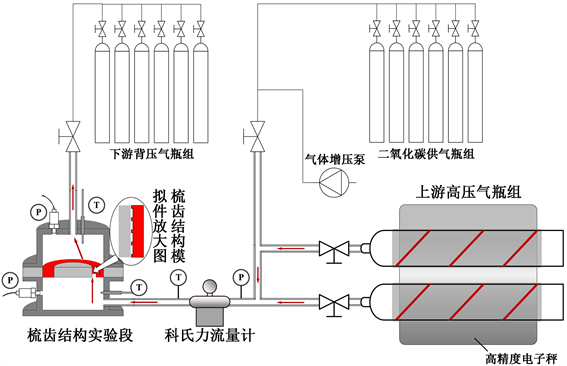Figure 1. Schematic diagram of experimental loop

2.2. 梳齿模拟件结构参数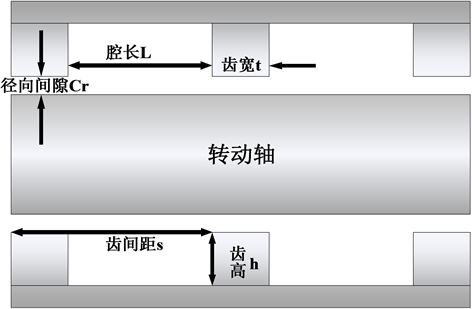Figure 2. Schematic diagram of labyrinth seal structureTable 1. Structural parameters of the labyrinth seal

3. 计算模型

3.1. 快速计算模型

3.1.1. Hodkinson计算模型 

Hodkinson后来在Egli方程的基础上进行修正，引入一个半经验系数代替Egli方程的动能携带系数，见式(1)，式(2)。Hodkinson考虑了气体射流结构的影响，假设齿顶的喷流的形状是一个小角度的锥形，其中一部分射流撞击在下游梳齿上使流体动能耗散，部分没有耗散的动能被带入到下一个腔室中。研究中假设气体喷射角度是密封结构的函数。Hodkinson给出了以下泄漏量计算半经验公式：

$\stackrel{˙}{m}={\mu }_{i}\frac{A\ast {P}_{in}}{\sqrt{R\ast T}}\sqrt{\frac{1-{\left(\frac{{P}_{out}}{{P}_{in}}\right)}^{2}}{n-\mathrm{ln}\left(\frac{{P}_{out}}{{P}_{in}}\right)}}$ (1)

${\mu }_{i}=\sqrt{\frac{1}{1-\left(\frac{n-1}{n}\right)\ast \left(\frac{C{r}_{i}/{L}_{i}}{\left(C{r}_{i}/{L}_{i}\right)+0.02}\right)}}$ (2)

3.1.2. Vermes计算模型 

Vermes利用射流边界层理论推导了动能携带系数，对Martin方程进行了非绝热条件下的修正。式(3)即Martin方程原型，式(4)即考虑了梳齿结构中径向间隙，齿间距，齿宽等参数对动能携带系数的影响。

$\stackrel{˙}{m}={\mu }_{i}\frac{A\ast {P}_{in}}{\sqrt{R\ast T}}\sqrt{\frac{1-{\left(\frac{{P}_{out}}{{P}_{in}}\right)}^{2}}{n-\mathrm{ln}\left(\frac{{P}_{out}}{{P}_{in}}\right)}}$ (3)

${\mu }_{i}=\sqrt{\frac{1}{1-{\alpha }_{i}}}$ 其中 ${\alpha }_{i}=\frac{8.52}{\frac{{s}_{i}-{t}_{i}}{C{r}_{i}}+7.23}$ (4)

3.2. 迭代式计算模型

3.2.1. De Saint Venant计算模型 

Vennard和Street  推导出一个一维流动的能量守恒方程，其一维模型如图4所示，同时获得了欧拉方程(5)。将方程中高度势能项gdz忽略掉，并且将等熵关系方程(6)代入到欧拉方程中得到速度项表达式(8)。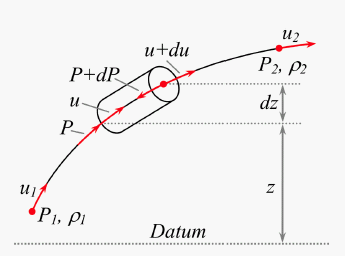Figure 4. Conservation of differential fluid energy on a streamline 

$\frac{\text{d}P}{\rho }+u\cdot \text{d}u+g\cdot \text{d}z=0$ (5)

$\frac{{P}_{i}}{{\rho }_{i}^{\gamma }}=\frac{{P}_{i+1}}{{\rho }_{i+1}^{\gamma }}$ (6)

$\frac{{u}_{i}^{2}-{u}_{cav}^{2}}{2}={\int }_{{P}_{i+1}}^{{P}_{i}}\frac{\text{d}P}{\rho }=\frac{{P}_{i}}{{\rho }_{i}}\cdot \frac{\gamma }{\gamma -1}\cdot \left[1-{\left(\frac{{P}_{i+1}}{{P}_{i}}\right)}^{\frac{\gamma }{\gamma -1}}\right]$ (7)

${u}_{i}=\sqrt{\frac{2\cdot \gamma }{\gamma -1}\cdot \frac{{P}_{i}}{{\rho }_{i}}\cdot \left[1-{\left(\frac{{P}_{i+1}}{{P}_{i}}\right)}^{\frac{\gamma }{\gamma -1}}\right]}$ (8)

${\stackrel{˙}{m}}_{i}={\rho }_{i}\cdot {A}_{i}\cdot {u}_{i}$ (9)

${\stackrel{˙}{m}}_{i}=\frac{{P}_{i}\cdot {A}_{i}}{\sqrt{R\cdot {T}_{i}}}\cdot \sqrt{\frac{2\cdot \gamma }{\gamma -1}\cdot \left[{\left(\frac{{P}_{i+1}}{{P}_{i}}\right)}^{\frac{2}{\gamma }}-{\left(\frac{{P}_{i+1}}{{P}_{i}}\right)}^{\frac{\gamma +1}{\gamma }}\right]}$ (10)

3.2.2. Neumann计算模型 

Neumann建立了一个泄漏量的经验公式(12)。包含了半经验流量系数Cf和动能携带系数μ，如式(13)(14)。Cf是利用气体方程计算出来，解释了流体流过梳齿的进一步收缩。

$\stackrel{˙}{m}=C{f}_{i}\ast {\mu }_{i}\ast {A}_{i}\sqrt{\frac{{P}_{i}^{2}-{P}_{i+1}^{2}}{RT}}$ (12)

$C{f}_{i}=\frac{\pi }{\pi +2-5{\beta }_{i}+{\beta }_{i}^{2}}$ 其中 ${\beta }_{i}={\left(\frac{{P}_{i}}{{P}_{i+1}}\right)}^{\frac{\gamma -1}{\gamma }}-1$ (13)

${\mu }_{i}=\sqrt{\frac{n}{n\ast \left(1-{\alpha }_{i}\right)+{\alpha }_{i}}}$ 其中 ${\alpha }_{i}=1-\frac{1}{{\left(1+\frac{16.6\ast C{r}_{i}}{{s}_{i}}\right)}^{2}}$

4. 实验数据与现有计算模型比较结果

4.1. 不同压比参数下模型评估Figure 5. Comparison of models under different pressure ratio parameters

4.2. 不同上游滞止压力参数下模型评估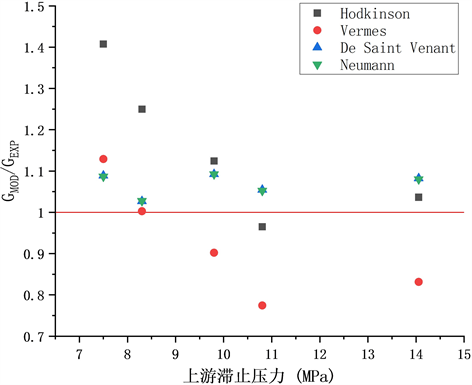Figure 6. Comparison of models under different upstream stagnation pressure parameters

4.3. 不同腔长与径向间隙比参数下模型评估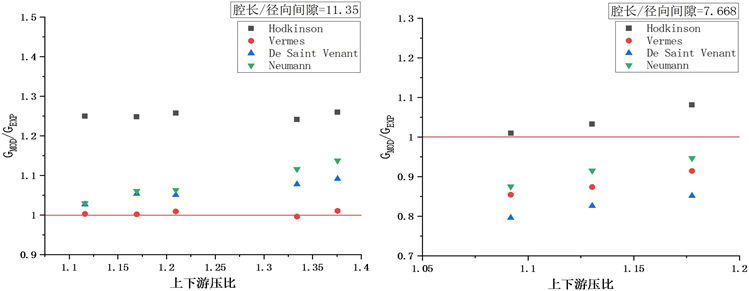(a) (b)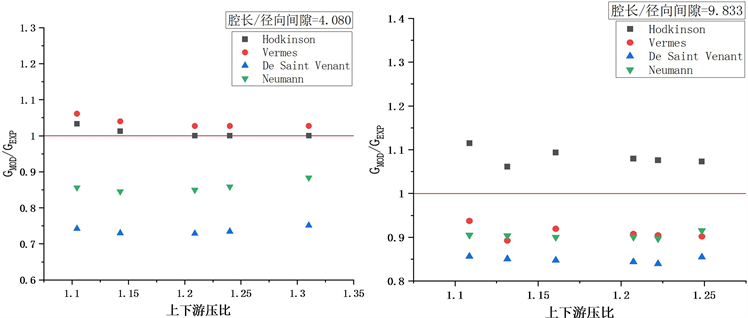(c) (d)

Figure 7. Comparison of models under different L/Cr

5. 结论

1) 压比约为1.1时，De Saint Venant模型与Neumann模型适用性较好，误差均不超过9.3%。

2) 在压比超过1.1情况下，上游滞止压力超过10.8 MPa范围内Hodkinson模型误差不超过4%。Vermes模型在上游滞止压力低于10.8 MPa范围内误差不超过12.9%。

3) 在上游滞止压力条件8.3 MPa条件下，腔长与径向间隙比为11.35时，Vermes模型预测与实验值契合度较高，误差不超过1.1%。腔长与径向间隙比小于11.35时，Hodkinson模型预测能力最优，误差不超过11.5%。

 USDoE (2002) A Technology Roadmap of Generation IV Nuclear Energy Systems. Proceedings of Nuclear Energy Research Advisory Committee and the Generation IV International Forum, Issy-les-Moulineaux.

 Dostal, V., Driscoll, M.J. and Hejzlar, P. (2004) A Supercritical Carbon Dioxide Cycle for Next Generation Nuclear Reactors. MIT-ANP-TR-100.

 Pidaparti, S.R. (2013) A Computational Study on the Leakage of Supercritical Carbon Dioxide through Labyrinth Seals. Texas A&M University, College Station.

 Martin (1908) H. M. Labyrinth Packings. Engineering, 35-36.

 Egli, A. (1935) The Leakage of Steam through Labyrinth Seals. Transactions of the ASME, 57, 115-122.

 Hodkinson, B. (1939) Estimation of the Leakage through a Labyrinth Gland. Proceedings of the Institution of Mechanical Engineers, 141, 283-288.
https://doi.org/10.1243/PIME_PROC_1939_141_037_02

 Vermes, G. (1961) A Fluid Mechanics Approach to the Labyrinth Seal Leakage Problem. ASME Transactions—Journal of Engineering for Power, 83, 161-169.
https://doi.org/10.1115/1.3673158

 Zimmerman, H. and Wolff, K.H. (1987) Comparison between Empirical and Numerical Labyrinth Flow Correlations. ASME 87-GT-86.
https://doi.org/10.1115/87-GT-86

 Mohamed, A. and Eldin, G. (2007) Leakage and Rotordynamic Effects of Pocket Damper Seals and See-Through Labyrinth Seals. Texas A&M University, College Station.

 Childs, D.W. and Scharrer, J. (1988) Theory versus Experiment for the Rotordynamic Coefficient of Labyrinth Gas Seals: Part II—A Comparison to Experiment. Journal of Vibration, Acoustics, Stress and Reliability in Design, 110, 281-287.
https://doi.org/10.1115/1.3269514

 Esser, D. and Kazakia, J. Y., 1995 Air Flow in Cavities of Labyrinth Seals. International Journal of Engineering Science, 33, 2309-2326.
https://doi.org/10.1016/0020-7225(95)00072-6

 Vennard, J.K. and Street, R.L. (1982) Elementary Fluid Mechanics. John Wiley& Sons, New York.

Top